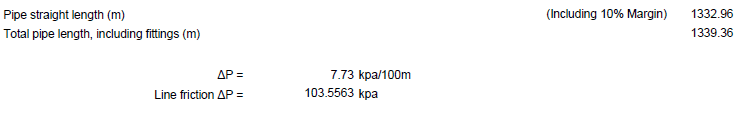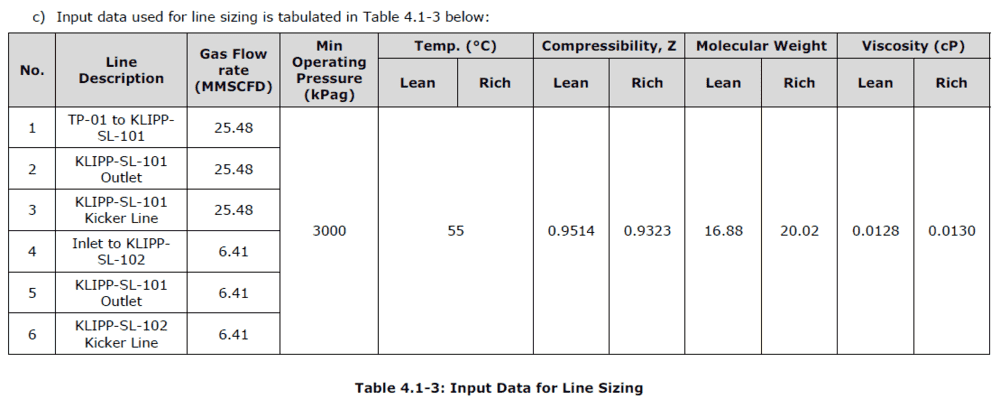# Length of a Pipeline

Hi,

I want to ask how to calculate the length of pipeline..From what i have searched, one of the way to calculate it is from the equation of pressure drop. However, it seems i dont have info about the pipe length and pressure drop (it means i need to calculate it). Is it possible to calculate the pipe length without those two info?

As in the attachment are the type of parameter may related to calculate the length of pipeline

Regard,
Mirul

## Answers and Replies

Chestermiller
Mentor
What are you actually trying to calculate, and what are you given?

•jim mcnamara
berkeman
Mentor
how to calculate the length of pipeline
Usually you are given the length of the pipeline as one of the first specifications. How can you not know how far you need to pipe your product?

•jim mcnamara
What are you actually trying to calculate, and what are you given?

i want to calculate the line friction and i believe in order to calculate it i need the length of pipeline.On the other side, the input are gas flowrate, pressure, temperature, molecular weight, compressibility and viscosity. However, i really doubt molecular weight, compressibility and viscosity are input and need to be calculate too.Chestermiller
Mentor
i want to calculate the line friction and i believe in order to calculate it i need the length of pipeline.
View attachment 266269

On the other side, the input are gas flowrate, pressure, temperature, molecular weight, compressibility and viscosity. However, i really doubt molecular weight, compressibility and viscosity are input and need to be calculate too.
View attachment 266270
What is your definition of the "pipe friction", the shear stress at the wall? Or is it the pressure gradient times the length of pipe?